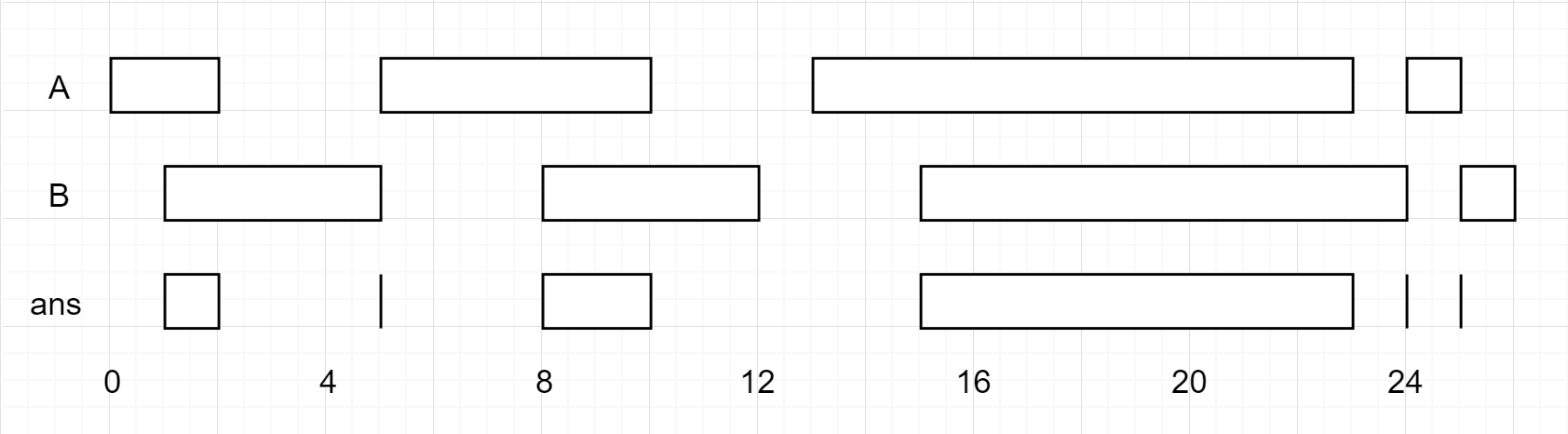0986. Interval List Intersections

# 986. Interval List Intersections#

## 题目 #

Given two lists of closed intervals, each list of intervals is pairwise disjoint and in sorted order.

Return the intersection of these two interval lists.

(Formally, a closed interval [a, b] (with a <= b) denotes the set of real numbers x with a <= x <= b. The intersection of two closed intervals is a set of real numbers that is either empty, or can be represented as a closed interval. For example, the intersection of [1, 3] and [2, 4] is [2, 3].)

Example 1:``````Input: A = [[0,2],[5,10],[13,23],[24,25]], B = [[1,5],[8,12],[15,24],[25,26]]
Output: [[1,2],[5,5],[8,10],[15,23],[24,24],[25,25]]
Reminder: The inputs and the desired output are lists of Interval objects, and not arrays or lists.
``````

Note:

• 0 <= A.length < 1000
• 0 <= B.length < 1000
• 0 <= A[i].start, A[i].end, B[i].start, B[i].end < 10^9

Note: input types have been changed on April 15, 2019. Please reset to default code definition to get new method signature.

## 代码 #

``````
package leetcode

/**
* Definition for an interval.
* type Interval struct {
*	   Start int
*	   End   int
* }
*/
func intervalIntersection(A []Interval, B []Interval) []Interval {
res := []Interval{}
for i, j := 0, 0; i < len(A) && j < len(B); {
start := max(A[i].Start, B[j].Start)
end := min(A[i].End, B[j].End)
if start <= end {
res = append(res, Interval{Start: start, End: end})
}
if A[i].End <= B[j].End {
i++
} else {
j++
}
}
return res
}

``````